﻿ Predictability of the Electrical Conductivity of In situ Sea Water as a Function of Its pH

### Predictability of the Electrical Conductivity of In situ Sea Water as a Function of Its pH

C. I. Nwoye, I. E. Nwosu, S. O. Nwakpa, J. U. Odo, S. E. Ede, N. E. IdenyiOPEN ACCESSPEER-REVIEWED

## Predictability of the Electrical Conductivity of In situ Sea Water as a Function of Its pH

C. I. Nwoye1,, I. E. Nwosu2, S. O. Nwakpa1, J. U. Odo1, S. E. Ede3, N. E. Idenyi4

1Department of Metallurgical and Materials Engineering, Nnamdi Azikiwe University, Awka, Nigeria

2Department of Environmental Technology, Federal University of Technology, Owerri, Nigeria

3Department of Metallurgical and Materials Engineering, Enugu State University of Science & Technology, Enugu, Nigeria

4Department of Industrial Physics Ebonyi State University, Abakiliki, Nigeria

### Abstract

The paper presents an empirical analysis which predicts the electrical conductivity of in situ sea water as function of its pH. Response coefficient of the sea water electrical conductivity ζ to the influence of its pH ϑ was evaluated to ascertain the reliability of the highlighted dependence. A univariate model was derived, validated and used for the predictive evaluation of the in situ sea water electrical conductivity. The validity of the model; ζ=exp[ϑ0.963] was rooted on the core model expression lnζ = ϑ0.963 where both sides of the expression are correspondingly approximately equal. Standard errors incurred in predicting the sea water electrical conductivity for each value of the sea water pH considered as obtained from experiment, derived model and regression model-predicted results were 0.5233, 1.2127 and 0.0027% respectively. Furthermore the correlation between sea water electrical conductivity and its pH as obtained from experiment, derived model and regression model were all > 0.9. The maximum deviation of the model-predicted sea water electrical conductivity (from experimental results) was less than 18%. This translated into over 82% operational confidence for the derived model as well as over 0.82 response coefficients for the dependence of in situ sea water electrical conductivity on its pH.

### At a glance: Figures

12
Prev Next

• Nwoye, C. I., et al. "Predictability of the Electrical Conductivity of In situ Sea Water as a Function of Its pH." Journal of Ocean Research 2.2 (2014): 23-27.
• Nwoye, C. I. , Nwosu, I. E. , Nwakpa, S. O. , Odo, J. U. , Ede, S. E. , & Idenyi, N. E. (2014). Predictability of the Electrical Conductivity of In situ Sea Water as a Function of Its pH. Journal of Ocean Research, 2(2), 23-27.
• Nwoye, C. I., I. E. Nwosu, S. O. Nwakpa, J. U. Odo, S. E. Ede, and N. E. Idenyi. "Predictability of the Electrical Conductivity of In situ Sea Water as a Function of Its pH." Journal of Ocean Research 2, no. 2 (2014): 23-27.

 Import into BibTeX Import into EndNote Import into RefMan Import into RefWorks

### 1. Introduction

The growing applicability of sea water has raised the need for intensive research and development geared towards correlating its physical properties. Sea water is known to contain dissolved salts. These salts are compounds like sodium chloride, magnesium sulfate, potassium nitrate, sodium bicarbonate which dissolve into ions.

Research  has shown that the properties of seawater that are most important for the dynamics of the circulation and the ocean-atmosphere climate system are (i) its large density (around a thousand times that of air) ≈ 1025kg m-3. (ii) its temperature and salinity, which modifies its density (cold and/or salty water is more dense than warm and/or fresh water). (iii) its specific heat per unit volume (J K-1 m-3) is 4000 times greater than that of air.

It was reported  that the density of seawater is a nonlinear function of salinity, temperature, and pressure;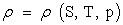(1)

The report also revealed that seawater is slightly compressible (its volume decreases under pressure), thus its density increases with pressure. The researcher stated that the equation of state can be approximated using a linear function of temperature and salinity;(2)

Further findings from the research  indicate that both the thermal expansion and haline contraction coefficients are functions of temperature and salinity.

Also the coefficients of thermal expansion and haline contraction change with pressure as well. The research  also show that on increasing the pressure, the in situ temperature increases (adiabatic warming) resulting to increase in the in situ density. On the other hand, removing the effects of the pressure on temperature and density, yields the potential temperature and density, which is the temperature and density that is only relevant to the dynamics of the circulation.

Research  has shown that before sea water pH is calculated on the “total” hydrogen scale, the potentiometric electrode is calibrated with sea water buffers 2-amino-2-hydroxymethyl-1,3-propanedion (tris) and 2-amino-2-methy-1,3-propanediol (bis).. Earlier work  revealed that the electrode slope and an isoelectric point is computed from the mv output when the electrode is immersed in the sea water buffers as described by the scientist. Based on the foregoing, the pH of sea water is calculated using the electrode slope and isoelectric point and sample temperature.

Improvement of the precision of time-series pH measurement data requires evaluation of the spectrophotometric methods for pH measurements . Similarly, other researchers  have measured the pH of sea water using spectrophotometric techniques.

Studies [6, 7] have indicated that the use of electrical conductivity measurement to estimate the ionic content of sea water led to the development of the Practical Salinity Scale in 1978 (PSS-78).

Limnologists  have used electrical conductivity or ‘‘reference conductivity,’ as a proxy for salinity called. This measurement may be corrected for temperature effects, and is usually expressed in units of μS/cm.

The electrical conductivity of seawater is a function of salinity and temperature . The research revealed precisely that conductivity and temperature measurements can be used to infer salinity which is measured in terms of the practical salinity unit; nearly equivalent to g/kg.

A successful attempt  has been made to measure and record the electrical conductivity of sea water as a function of depth from a moving ship. This work implies that the electrical conductivity of the sea water varies with depth.

The aim of this research is to predict the electrical conductivity of in situ sea water as a function of its pH. An empirical model will be derived, validated and used for the predictive analysis. It is expected that if the work is successful, the model will be applied for predicting the electrical conductivity of any water providing its pH is within the pH boundary condition in this work.

### 2. Materials and Methods

Materials used for the experiment are sea water (collected from in situ sea located at Okirika in Port Harcourt, Nigeria), flying boat, beakers, conical flasks and electrical conductivity probe (XCTD Probe). The other materials used were analytical digital weighing machine (Mettle 4900), and pH meter (SeaFETTM Ocean pH Sensor).

2.1. Specimen collection and measurement of electrical conductivity

Flying boat was used in plying around the in situ sea water. The specimens were collected within a period of six months (January-June 2014) to accommodate and integrate any change in the salinity and pH of the sea water. Seven days (a week) were randomly picked in each month for specimen collection, making a total active collection time 6weeks. For each seven days (a week) of specimen collection, 60 samples of the sea water were collected from 60 different points in the sea. The pH of these sea water samples were respectively measured (at the collection site) using SeaFETTM Ocean pH Sensor and average value recorded on per week basis. Furthermore, the electrical conductivity of each of the sea water samples (whose pH was already measured) was also measured at the collection site using XCTD Probe and then recorded against its corresponding pH value. It is pertinent to state that the measurement of both sea water pH and electrical conductivity were simple since their respective equipment are handy and have ease of handling.

### 3. Results and Discussion

3.1. Variation of the electrical conductivity of in situ sea water with its pH

Table 1 shows that electrical conductivity of the sea water increases with increase in its pH. Since the pH of salt and water co-produced following neutralization reaction is ≈ 7, Table 1 indicates that the electrical conductivity of the sea water will increase with increase in saltiness of the water, that is pH approaching 7. Based on the foregoing, the electrical conductivity of the in situ sea water will increase with increase in its pH within a pH range: 6.57– 6.80.

Effect of Seasonal Variation on the Sea water Electrical Conductivity as Function of the sea water pH

It is strongly believed that the electrical conductivity of in situ sea water as a function of its pH would be affected by seasonal variation. This is because the temperature of the in situ sea water varies at different periods of the year. Variation in the temperature of the in situ sea water affects the rate of liberation of CO2 from carbonates dissolved in the water. It therefore follows that since CO2 is an acid forming gas, the sea water pH would likely be affected by the exist of CO2. Furthermore, variation in temperature affects the solubility of salts in the sea water and invariably affects the pH.

Computational analysis of generated experimental data shown in Table 1, gave rise to Table 2 which indicate that;(3)

Introducing the value of N into equation (3) reduces it to;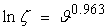(4)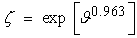(5)

N = 0.963; an empirical constant (determined using C-NIKBRAN 

(ζ ) = In situ sea water electrical conductivity (μS/cm)

(ϑ) = pH of in situ sea water

#### Table 1. Variation of electrical conductivity of in situ sea water ζ with its pH

The derived model is equation (5). Computational analysis of Table 1 gave rise to Table 2. The derived model is univariate in nature since it is composed of just one input process parameter: pH of sea water. This implies that the predicted in situ sea water electrical conductivity is dependent on pH of the sea water

Boundary and Initial Conditions

Consider in situ sea water, interacting with some corrosion-induced agents. The sea water is assumed to be affected by unwanted dissolved gases and minerals. Range of the sea water electrical conductivity and pH considered: 477.6 – 480.5 and 6.57 – 6.80 respectively.

The boundary condition is: aerobic environment, since the atmosphere contains oxygen

3.1.2 Model Formulation
3.1.3. Model Validity

The validity of the model is strongly rooted on equation (4) (core model equation) where both sides of the equation are correspondingly approximately equal. Table 2 also agrees with equation (4) following the values of lnζ and ϑ0.963 evaluated from the experimental results in Table 1. Furthermore, the derived model was validated by comparing the sea water electrical conductivity predicted by the model and that obtained from the experiment. This was done using various analytical techniques.

3.1.3.1. Statistical analysis

Standard Error (STEYX)

The standard errors incurred in predicting the sea water electrical conductivity for each value of its pH considered as obtained from experiment and derived model were 0.5233 and 1.2127% respectively. The standard error was evaluated using Microsoft Excel version 2003.

Correlation

The correlation coefficient between sea water electrical conductivity and its pH were evaluated (using Microsoft Excel Version 2003) from results of the experiment and derived model. These evaluations were based on the coefficients of determination R2 shown in Figs. 1and 2.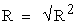(6)
Figure 1. Coefficient of determination between sea water electrical conductivity and its pH as obtained from the experiment
Figure 2. Coefficient of determination between sea water electrical conductivity and its pH as predicted by derived model

#### Table 3. Comparison of the correlations between sea water electrical conductivity and its pH as evaluated from experimental (ExD) and derived model (MoD) predicted results

The evaluated correlations are shown in Table 3. These evaluated results indicate that the derived model predictions are significantly reliable and hence valid considering its proximate agreement with results from actual experiment.

3.1.3.2. Graphical Analysis

Graphical analysis of Figure 3 shows very close magnitudes of bar heights from derived model and experiment. It is strongly believed that the degree of dimensional closeness of these bars is indicative of the proximate agreement between ExD and MoD predicted results.

Figure 3. Comparison of the sea water electrical conductivities (relative to its pH) as obtained from experiment and derived model

3.1.3.3. Comparison of derived model with standard model

The validity of the derived model was also verified through application of the regression model (ReG) (Least Square Method using Excel version 2003) in predicting the trend of the experimental results. Comparative analysis of Figure 4 shows very close magnitudes of bar heights which precisely translated into significantly similar trend of data point’s distribution for experimental (ExD), derived model (MoD) and regression model-predicted (ReG) results of sea water electrical conductivity.

Also, the evaluated correlation (from Figure 4) between sea water electrical conductivity and its pH for results obtained from regression model was 1.0000. These values are in proximate agreement with both experimental and derived model-predicted results. The standard error incurred in predicting sea water electrical conductivity for each value of sea water pH considered as obtained from regression model was 0.0027 %.

Figure 4. Comparison of the sea water electrical conductivities (relative to its pH) as obtained from experiment, derived model and regression model

3.1.3.4. Deviational Analysis

Comparative analysis of the sea water electrical conductivity precisely obtained from experiment and derived model shows that the model-predicted values deviated from experimental results. This was attributed to the fact that some process conditions involved in pH and electrical conductivity measurement which affected generated results were not considered during the model formulation. This necessitated the introduction of correction factor, to bring the model-predicted seawater electrical conductivity to those of the corresponding experimental values.

The deviation Dv, of model-predicted seawater electrical conductivity from the corresponding experimental result was given by(7)

Where

ζExD and ζMoD are sea water electrical conductivity evaluated from experiment and derived model respectively.

Figure 5. Variation of model-predicted sea water electrical conductivity with associated deviation from experimental results

Figure 5 shows that the least and highest magnitudes of deviation of the model-predicted sea water electrical conductivity (from the corresponding experimental values) are – 1.56 and + 17.30%. It could be seen from Figure 5 that these deviations correspond to sea water electrical conductivity: 471.02 and 563.63 μS/cm and sea water pH: 6.6 and 6.8 respectively.

Analysis of Figure 5 also shows that the maximum deviation of model-predicted sea water electrical conductivity from the experimental results was less than 18%. This translated into over 82% operational confidence and response level for the derived model as well as over 0.82 response coefficients for the dependence of in situ sea water electrical conductivity on its pH.

Correction factor, Cf to the model-predicted sea water electrical conductivity was given by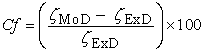(8)

#### Table 4. Variation of correction factor with sea water electrical conductivity and pH

Analysis of Table 4 in comparison with Figure 5 indicates that the evaluated correction factors are negative of the deviation as shown in equations (7) and (8).

The correction factor took care of the negligence of some process conditions involved in sea water pH and electrical conductivity measurement, which actually affected generated results. The model predicted results deviated from those of the experiment because these conditions were not considered during the model formulation. Introduction of the corresponding values of Cf from equation (8) into the model gives exactly the corresponding experimental sea water electrical conductivity.

Table 4 shows that the least and highest magnitudes of correction factor to the model-predicted sea water electrical conductivity are + 1.56 and – 17.37%. Since correction factor is the negative of deviation (equations (7) and (8)), Table 4, Figure 1 and Figure 2 indicate that these correction factors correspond to sea water electrical conductivity: 471.02 and 563.63 μS/cm and sea water pH: 6.6 and 6.8 respectively.

It is important to state that the deviation of model predicted results from that of the experiment is just the magnitude of the value. The associated sign preceding the value signifies that the deviation is a deficit (negative sign) or surplus (positive sign).

### 4. Conclusion

An empirical analysis predicted the electrical conductivity of in situ sea water as a function of its pH. The validity of the univariate model derived, validated and used for the predictive evaluation of the in situ sea water electrical conductivity was rooted on the core model expression lnζ = ϑ0.963 where both sides of the expression are correspondingly approximately equal. Standard errors incurred in predicting the sea water electrical conductivity for each value of the sea water pH considered as obtained from experiment, derived model and regression model-predicted results were 0.5233, 1.2127 and 0.0027% respectively. Furthermore the correlation between sea water electrical conductivity and its pH as obtained from experiment, derived model and regression model were all > 0.9. The maximum deviation of the model-predicted seawater electrical conductivity (from experimental results) was less than 18%. This translated into over 82% operational confidence for the derived model as well as over 0.82 response coefficients for the dependence of in situ sea water electrical conductivity on its pH.

Limitations on predicting in situ sea water electrical conductivity as a function of sea water pH.

The limitations of predicting in situ sea water electrical conductivity as a function of the sea water pH could be surmounted by measuring the in situ sea water pH on a daily basis all through the year and determining the average value. This should be carried out for 6 or 7 years.

Based on the foregoing, reliable values of the in situ sea water electrical conductivity would be determined because any drop in sea water pH (at a particular period of the year when the temperature changes) as a result of variation in sea water temperature will be upgraded at periods of the year when the temperature is brought to normalcy. This implies that the year round variations will cancel out.

### References

  Wetzel, R. G. (2011). Limnology: Lake And River Ecosystem, 3rd ed. Academic press ISBN: 978-0-12-744760-5.In article  Dickson, A. G. (1991). pH Buffers for Sea Water Media Based on the Total Hydrogen Ion Concentration Scale. Deep – Sea Research.In article  Grasshoff, K. (1983). Determination of pH. In: Methods of Seawater Analysis, K. Grasshoff and K. Kremling, editors, Verlag- Chemie, pp. 85-97.In article  Byrne, R. H. (2011). Device for In Situ Calibrated Potentiometric pH Measurement US Patent, Publication no. US 8071031 B1.In article  Clayton, T. D and Byrne, R. H. (1993).Spectrophotometric Seawater pH Determination. www.ocean-sci.net/../os-7-597-2011.pdfIn article  UNESCO (1981).The Practical Salinity Scale 1978 and the International Equation of State of Sea water 1980. UNESCO Technical Paper Marine Science, Vol. 36. www.jodc.go.jp/../UNESCO_Tech.htmIn article  UNESCO (1981). Background papers and supporting Data on the Practical salinilty Scale 1978. UNESCO Technical Paper Marine Science, Vol. 37. www.jodc.go.jp/../UNESCO_Tech.htmIn article  Leif, T. Physical Characteristics of the Ocean EESS 146B/246B pangea.stanford.edu/courses/EESS146B…In article  Benneth, D. F. (1964).Measuring and Recording the Electrical Conductivity of Sea Water as a Function of Depth. US Patent Publication No. 3147431A.In article  Nwoye, C. I. (2008). C-NIKBRAN Data Analytical Memory (Software).In article PubMed